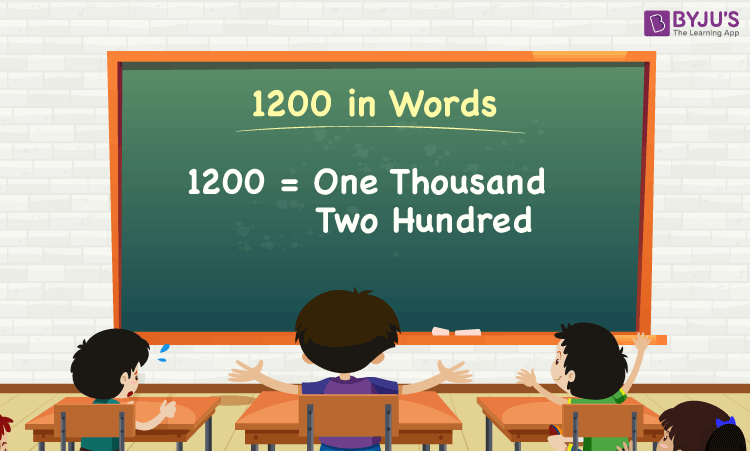# 1200 in Words

1200 in words is One Thousand Two Hundred. The number name of 1200 is written using the ones, tens, hundreds and thousands places of the number. Hence, we can say that the place value chart is very helpful in writing the number 1200 in words. For instance, if you bought a shirt worth Rs. 1200, you can write, “I have bought a shirt worth Rs. One Thousand Two Hundred”.

 1200 in Words: One Thousand Two Hundred

Here, we are going to learn the spelling of 1200 in words, and the procedure for writing the number 1200 in detail with many examples.

## How to Write 1200 in Words?To write 1200 in words, first, identify the digit’s place value of the given number. Hence, for the number 1200,

The digit in 1’s place is 0.

The digit in 10’s place is 0.

The digit in 100’s place is 2.

The digit in 1000’s place is 1.

Thus, the place value chart for the number 1200 is:

 Thousands Hundreds Tens Ones 1 2 0 0

Hence, 1200 in words is One Thousand Two Hundred.

### Examples

Example 1:

Compute the value of 1500 – 300. Express the value in words.

Solution:

The arithmetic expression given here is 1500 – 300

⇒ 1500 – 300 = 1200

So the value of 1500 – 300 is 1200.

Hence, 1200 in words is one thousand two hundred.

Example 2:

Rahul bought the notebooks which cost one thousand two hundred rupees. Along with that, he has purchased pens that cost two hundred rupees. Find the total cost, and express it in words.

Solution:

The cost of notebooks = One thousand two Hundred = 1200

The cost of pens = Two thousand = 200

Therefore, the total cost = Cost of notebooks + Cost of pens

= 1200 + 200

= 1400

Hence, the total cost of notebooks and pens is Rs. 1400.

Therefore, the total cost that Rahul spent on purchasing the notebooks and pen is one thousand four hundred rupees.

Keep visiting BYJU’S – The Learning App, and download the app to learn all Maths concepts easily by exploring more learning videos

## Frequently Asked Questions on 1200 in Words

Q1

### Write 1200 in words.

1200 in words is One Thousand Two Hundred.

Q2

### Simplify the value 2000 – 800, and express it in words.

Simplifying 2000 – 800, we get 1200. Hence, 1200 in words is one thousand two hundred.

Q3

### Convert one thousand two hundred in numbers.

One thousand two hundred in numbers is 1200.

Q4

### Is the number 1200 an even number?

Yes, the number 1200 is an even number.

Q5

### Is 1200 a perfect cube?

No, 1200 is not a perfect cube, as it cannot be expressed as the product of three equal integers.

Test your Knowledge on 1200 in Words